## How to calculate gear ratio? There is 4 easy ways to help you.

In mechanical engineering, a gear ratio is a direct measure of the ratio of the rotational speeds of two or more interlocking gears. As a general rule, when dealing with two gears, if the driving gear (the one directly receiving rotational force from the engine, motor, etc.) is bigger than the driven gear, the latter will turn more quickly, and vice versa. We can express this basic concept with the formula Gear ratio = T2/T1, where T1 is the number of teeth on the first gear and T2 is the number of teeth on the second.

## Method 1 of 2: Finding the Gear Ratio of a Gear Train

### Two Gears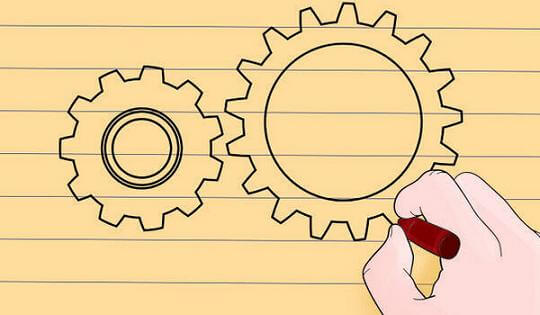To be able to determine a gear ratio, you must have at least two gears attached to each other — this is called a “gear train.” Usually, the first gear is a “drive gear” attached to the motor shaft and the second is a “driven gear” attached to the load shaft. There may also be any number of gears between these two to transmit power from the drive gear to the driven gear: these are called “idler gears.”

• For now, let’s look at a gear train with only two gears in it. To be able to find a gear ratio, these gears have to be interacting with each other — in other words, their teeth need to be interconnected and one needs to be turning the other. For example purposes, let’s say that we have one small drive gear (gear 1) turning a larger driven gear (gear 2). We’re ready to proceed.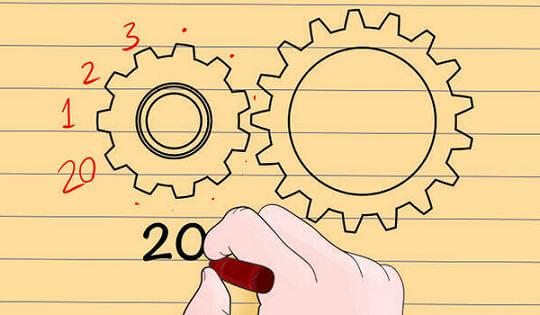2. Count the number of teeth on the drive gear.

One simple way to find the gear ratio between two interlocking gears is to compare the number of teeth (the little peg-like protrusions at the edge of the wheel) that they both have. Start by determining how many teeth are on the drive gear. You can do this by counting manually or, sometimes, by checking for this information labeled on the gear itself.

• For example purposes, let’s say that the smaller drive gear in our system has 20 teeth.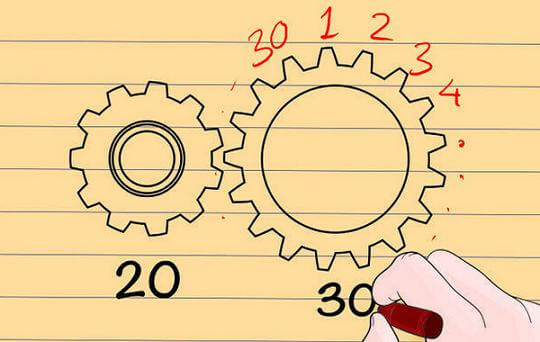3. Count the number of teeth on the driven gear.

Next, determine how many teeth are on the driven gear exactly as you did before for the drive gear.

• Let’s say that, in our example, the driven gear has 30 teeth.4. Divide one teeth count by the other.

Now that you know how many teeth are on each gear, you can find the gear ratio relatively simply. Divide the driven gear teeth by the drive gear teeth. Depending on your assignment, you may write your answer as a decimal, a fraction, or in ratio form (i.e., x : y).

• In our example, dividing the 30 teeth of the driven gear by the 20 teeth of the drive gear gets us 30/20 = 1.5. We can also write this as 3/2 or 1.5 : 1, etc.
• What this gear ratio means is that the smaller driver gear must turn one and a half times to get the larger driven gear to make one complete turn. This makes sense — since the driven gear is bigger, it will turn more slowly.

### More than Two Gears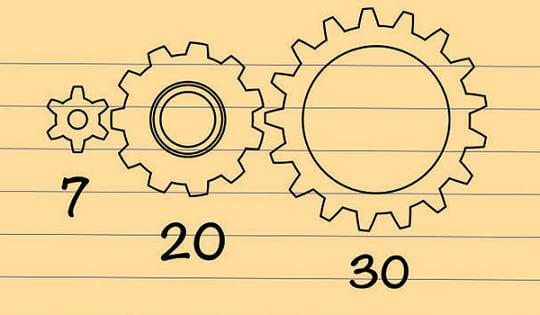1.Start with a gear train of more than two gears.

As its name suggests, a “gear train” can also be made from a long sequence of gears — not just a single driver gear and a single driven gear. In these cases, the first gear remains the driver gear, the last gear remains the driven gear, and the ones in the middle become “idler gears.” These are often used to change the direction of rotation or to connect two gears when direct gearing would make them unwieldy or not readily available.

• Let’s say for example purposes that the two-gear train described above is now driven by a small seven-toothed gear. In this case, the 30-toothed gear remains the driven gear and the 20-toothed gear (which was the driver before) is now an idler gear.2. Divide the teeth numbers of the drive and driven gears.

The important thing to remember when dealing with gear trains with more than two gears is that only the driver and driven gears (usually the first and last ones) matter. In other words, the idler gears don’t affect the gear ratio of the overall train at all. When you’ve identified your driver gear and your driven gear, you can find the gear ratio exactly as before.

• In our example, we would find the gear ratio by dividing the thirty teeth of the driven gear by the seven teeth of our new driver. 30/7 = about 4.3 (or 4.3 : 1, etc.) This means that the driver gear has to turn about 4.3 times to get the much larger driven gear to turn once.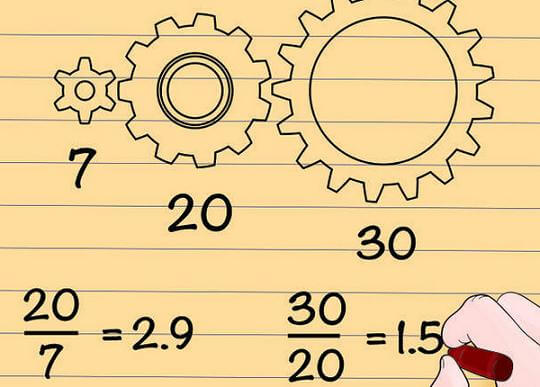3. If desired, find the gear ratios for the intermediate gears.

You can find the gear ratios involving the idler gears as well, and you may want to in certain situations. In these cases, start from the drive gear and work toward the load gear. Treat the preceding gear as if it were the drive gear as far as the next gear is concerned. Divide the number of teeth on each “driven” gear by the number of teeth on the “drive” gear for each interlocking set of gears to calculate the intermediate gear ratios.

• In our example, the intermediate gear ratios are 20/7 = 2.9 and 30/20 = 1.5. Note that neither of these are equal to the gear ratio for the entire train, 4.3.
• However, note also that (20/7) × (30/20) = 4.3. In general, the intermediate gear ratios of a gear train will multiply together to equal the overall gear ratio.

## Method 2 of 2: Making Ratio/Speed Calculations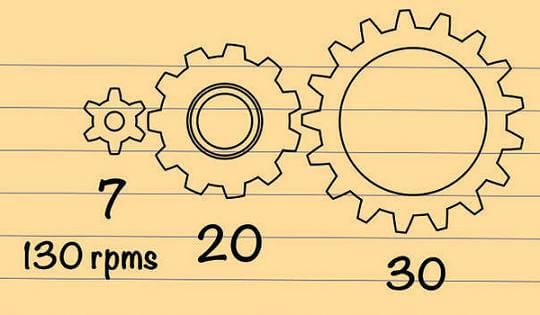1. Find the rotational speed of your drive gear.

Using the idea of gear ratios, it’s easy to figure out how quickly a driven gear is rotating based on the “input” speed of the drive gear. To start, find the rotational speed of your drive gear. In most gear calculations, this is given in rotations per minute (rpm), though other units of velocity will also work.

• For example, let’s say that in the example gear train above with a seven-toothed driver gear and a 30-toothed driven gear, the drive gear is rotating at 130 rpms. With this information, we’ll find the speed of the driven gear in the next few steps.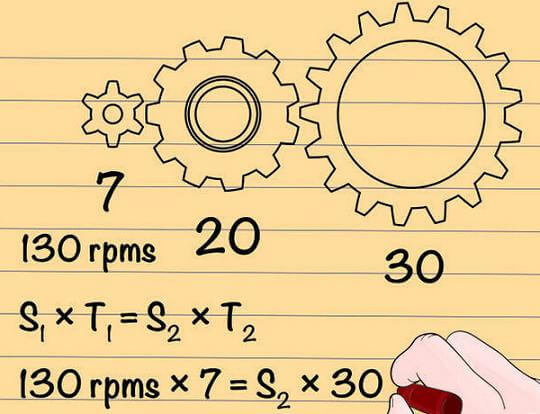2. Plug your information into the formula S1 × T1 = S2 × T2.

In this formula, S1 refers to the rotational speed of the drive gear, T1 refers to the teeth in the drive gear, and S2 and T2 to the speed and teeth of the driven gear. Fill in the variables until you have only one left undefined.

• Often, in these sorts of problems, you’ll be solving for S2, though it’s perfectly possible to solve for any of the variables. In our example, plugging in the information we have, we get this:
• 130 rpms × 7 = S2 × 30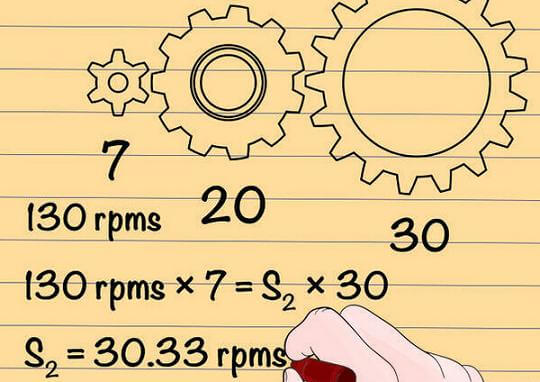3. Solve.

Finding your remaining variable is a matter of basic algebra. Just simplify the rest of the equation and isolate the variable on one side of the equals sign and you will have your answer. Don’t forget to label it with the correct units — you can lose points for this in schoolwork.
• In our example, we can solve like this:
• 130 rpms × 7 = S2 × 30
• 910 = S2 × 30
• 910/30 = S2
• 30.33 rpms = S2
• In other words, if the drive gear spins at 130 rpms, the driven gear will spin at 30.33 rpms. This makes sense — since the driven gear is much bigger, it will spin much slower.